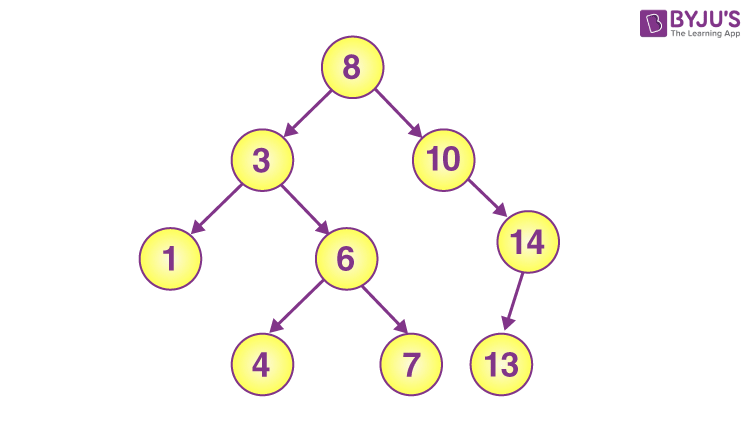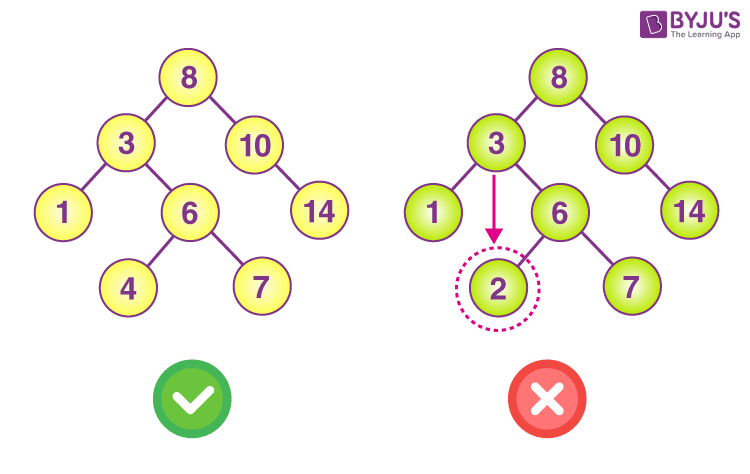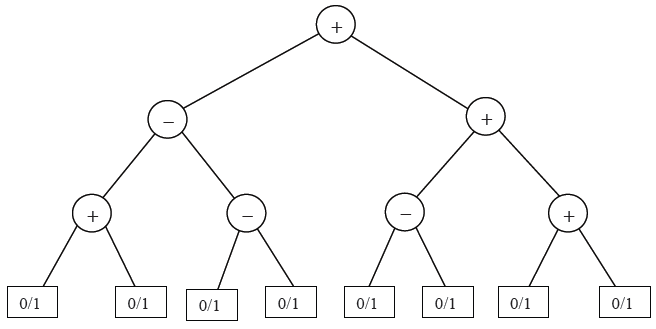# Binary Search Trees

Binary Search Tree is an important topic belonging to the famous chapter of computer science i.e. Data Structure. And, when it comes to a competitive examination like GATE, you have to read this whole topic quite deeply. In this article we have covered all the topics relevant to the linked list. We hope the notes for the CSE topics will help you understand this topic in a better way.

Topic of Contents

## What is a Binary Search Tree?

In computer science, a binary search tree is an important term. It is also known as an ordered or sorted binary tree. It contains a few properties like:

• The left subtree of a node includes only nodes with keys lesser than the node’s key.
• The right subtree of a node contains only nodes with keys greater than the node’s key.
• The left and right subtree each must also be a binary search tree.## Time Complexity In Binary Search Tree:

When it comes to the binary search tree, the time complexity is O(log n) time where n is the number of nodes in the tree. However, when it comes to the worst scenario, the time complexity for these operations is 0(n). In that condition the tree becomes unbalanced.

## Space Complexity In Binary Search Tree:

The space complexity of a binary search tree is O(n).

### Types of Traversals

Types of Traversals The Binary Search Tree can be traversed in the following ways:

1. Pre-order Traversal
2. In-order Traversal
3. Post-order Traversal

In preorder traversal, We start from the current node before going on to the left and right nodes.

In inorder traversal, we can traverse from the left node then hitting the current node and at the last right node.

In postorder traversal, we can start by traversing from the left child node before the right child node and then the current node.

### Video on Binary Tree Traversals Concept## Application Of Binary Search Tree

• Binary search tree is used to accomplish indexing and multi-level indexing.
• They are also capable of implementing various search algorithms.
• It aids in organizing a data stream.
• Self-balancing BSTs are used to implement TreeMap and TreeSet data structures.

## Operation in Binary Search Tree

1. Search
2. Insertion
3. Deletion

Search Operation: Through this operation, we can search the location of some particular element in a binary search tree.

Insertion Operation: Through this operation, we can add a node or element in the accurate place.

Deletion Operation: When we delete a specific node or element from the binary search tree, it is known as deletion operation.

• The binary search tree is fast in insertion and deletion etc when balanced.
• It is very effective and its code is easier as compared to the link lists.

• The shape of the tree depends upon the order of insertion and it can degenerate.
• In binary search trees, searching operations take a longer time.

Comparison Right Binary Tree Vs Wrong Binary Search Tree## Practice Problem – Binary Search Tree

Q. Let T be a binary search tree with 15 nodes. The minimum and maximum possible heights of T are:

Note: The height of a tree with a single node is 0

• (A) 4 and 15 respectively
• (B) 3 and 14 respectively
• (C) 4 and 14 respectively
• (D) 3 and 15 respectively

Q. The pre-order traversal of a binary search tree is given by 12, 8, 6, 2, 7, 9, 10, 16, 15, 19, 17, 20. Then the post-order traversal of this tree is:

• (A) 2, 6, 7, 8, 9, 10, 12, 15, 16, 17, 19, 20
• (B) 2, 7, 6, 10, 9, 8, 15, 17, 20, 19, 16, 12
• (C) 7, 2, 6, 8, 9, 10, 20, 17, 19, 15, 16, 12
• (D) 7, 6, 2, 10, 9, 8, 15, 16, 17, 20, 19, 12

Q. Consider the expression tree shown. Each leaf represents a numerical value, which can either be 0 or 1. Over all possible choices of the values at the leaves, the maximum possible value of the expression represented by the tree is ___.Keep learning and stay tuned to get the latest updates on GATE Exam along with GATE Eligibility CriteriaGATE 2023GATE Admit CardGATE Syllabus for CSE (Computer Science Engineering)GATE CSE NotesGATE CSE Question Paper, and more.

Also Explore,